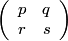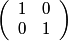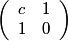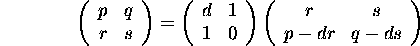#### Factorising a unimodular matrix

This program expresses a unimodular matrix A =or U0 =, with non-negative coefficients, as a product of the type P, U0P, PU0, or U0PU0, where P is a product of matrices of the form Uc =, c > 0.
The representation is unique. (See Kjell Kolden, Continued fractions and linear substitutions, Arch. Math. Naturvid. 50 (1949), 141-196.)

While r > 0 and s > 0, we repeatedly use the identitywhere d = min([p/r],[q/s]).

The process eventually stops, either because r = 0, in which case we reach UqU0,
or because s=0, in which case we reach Up.

This is a BCMATH translation of a BC program.

Enter p (≥ 0):
Enter q (≥ 0):
Enter r (≥ 0):
Enter s (≥ 0):